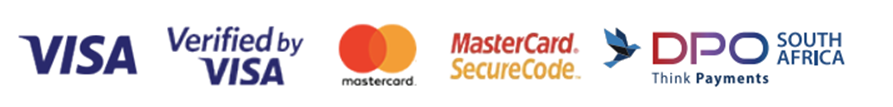﻿ Teacher Reference Books - Primary Maths - Singapore Maths
Showing 1 - 26 of 26 items
• ##### Teaching to Mastery Mathematics Bar Modelling: A Problem-Solving Tool
R625.00

Bar Modelling  - A Problem-solving Tool.  From reasearch to practice - an effective Singapore Math Strategy.  This is a professional development title.

• ##### Teaching to Mastery Mathematics: Teaching of Whole Numbers
R625.00

Teaching of Whole Numbers - From Research to Practice.  Based on the Singapore Maths Approach.  This is a professional development title.

• ##### Teaching to Mastery Mathematics: Teaching of Fraction
R625.00

Teaching of Fractions - From Research to Practice - Based on the Singapore Maths approach.  This is a professional development title.

• ##### Teaching to Mastery Mathematics: Teaching Of Decimals
R625.00

Teaching of Decimals - From Research to Practice.  Based on the Singapore Maths Approach.  This is a professional development title.

• ##### Teaching to Mastery Mathematics: Teaching Of Proprtional Reasoning
R625.00

Teaching of Proportional Reasonong - From Research to Practice.  Based on the Singapore Maths Approach.  This is a professional development title.

R340.00

• ##### Conquer Model Drawing For Lower Primary levels
R145.00

Conquer Model Drawing is a series of two books – Lower Primary and Upper Primary – specially conceived and developed to encapsulate the essence of mathematicalmodelling and harness students’ cognitive skills to solve word problems.

• ##### Conquer Model Drawing For Upper Primary levels
R145.00

Conquer Model Drawing is a series of two books – Lower Primary and Upper Primary – specially conceived and developed to encapsulate the essence of mathematicalmodelling and harness students’ cognitive skills to solve word problems.

• ##### Conquer Mathematics (The 4 Operations) Book 1
R275.00

The 4 Operations is specially designed to equip students with basic skills to solve questions involving addition, subtraction, multiplication and division of numbers up to 100. Book contains exercises that consist of multi-choice and short-answer questions. The exercises are diagnostic in nature.

• ##### Conquer Mathematics (Length, Time, and Money) Book 1
R275.00

Specifically designed to enhance students' proficiency in the mathematical concepts of time, length and money. Book contains exercises that consist of multi-choice and short-answer questions. The exercises are diagnostic in nature.

• ##### Conquer Mathematics (Shapes, Pattern, Picture Graphs) Book 1
R220.00

Designed to enhance students' proficiency in mathematics concepts of shapes, patterns and picture graphs. Book contains exercises that consist of multi-choice and short-answer questions. The exercises are diagnostic in nature.

• ##### Conquer Mathematics (The 4 Operations and Fractions) Book 2
R275.00

Designed to equip students with basic skills to solve questions involving the 4 operations and fractions. Book contains exercises that consist of multi-choice and short-answer questions. The exercises are diagnostic in nature.

• ##### Conquer Mathematics (Geometry and Picture Graphs) Book 2
R295.00

Designed to enhance students' proficiency in mathematics concepts of geometry and picture graphs. Book contains exercises that consist of multi-choice and short-answer questions. The exercises are diagnostic in nature.

• ##### Conquer Mathematics (Measurements, Volume, Time, Money) Book 2
R295.00

Designed to equip students with basic skills to solve questions involving measurements, volume, time and money. Book contains exercises that consist of multi-choice and short-answer questions. The exercises are diagnostic in nature.

• ##### Conquer Mathematics (The 4 Operations and Fractions) Book 3
R220.00

Designed to equip students with basic skills to solve questions involving the 4 operations and fractions. Book contains exercises that consist of multi-choice and short-answer questions. The exercises are diagnostic in nature.

• ##### Conquer Mathematics (Measurements, Volume, Time & Money) Book 3
R210.00

Designed to enhance students' proficiency in mathematics concepts of measurement, volume, time and money. Book contains exercises that consist of multi-choice and short-answer questions. The exercises are diagnostic in nature.

• ##### Conquer Mathematics (Geometry, Area, Perimeter and Bar Graphs) Book 3
R275.00

Designed to equip students with basic skills to solve questions involving geometry, area, perimeter and bar graphs. Book contains exercises that consist of multi-choice and short-answer questions. The exercises are diagnostic in nature.

• ##### Conquer Mathematics (Factors, Multiples, Fractions) Book 4
R220.00

Designed to equip students with basic skills to solve questions involving factors, multiples and fractions. Book contains exercises that consist of multi-choice and short-answer questions. The exercises are diagnostic in nature.

• ##### Conquer Mathematics (Decimals and Time) Book 4
R220.00

Designed to equip students with basic skills to solve questions involving decimals and time. Book contains exercises that consist of multi-choice and short-answer questions. The exercises are diagnostic in nature.

• ##### Conquer Mathematics (Geometry, Area and Perimeter, Money, Graphs) Book 4
R275.00

Designed to enhance students' proficiency in mathematics concepts of geometry, area, perimeter, money and graphs. Book contains exercises that consist of multi-choice and short-answer questions. The exercises are diagnostic in nature.

• ##### Conquer Mathematics (Fraction, Decimal, Percentage, Ratio) Book 5
R220.00

Designed to enhance students' proficiency in mathematics concepts of fractions, decimals, percentages and ratios. Book contains exercises that consist of multi-choice and short-answer questions. The exercises are diagnostic in nature.

• ##### Conquer Mathematics (Measurements, Area and Volume) Book 5
R220.00

Designed to enhance students' proficiency in mathematics concepts of measurement, area and volume. Book contains exercises that consist of multi-choice and short-answer questions. The exercises are diagnostic in nature.

• ##### Conquer Mathematics (Fraction, Percentage, Ratio) Book 6
R250.00

Designed to enhance students' proficiency in mathematics concepts of fractions, percentages and ratio. Book contains exercises that consist of multi-choice and short-answer questions. The exercises are diagnostic in nature.

• ##### 365 Daily Workout Maths Primary 3
R285.00

The 365 Daily Workout: Maths series provides systematic and regular practice for pupils.

• ##### 365 Daily Workout Maths Primary 4
R285.00

The 365 Daily Workout: Maths series provides systematic and regular practice for pupils.

• ##### 365 Daily Workout Maths Primary 5
R285.00

The 365 Daily Workout: Maths series provides systematic and regular practice for pupils.

Showing 1 - 26 of 26 items